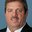Question-and-Answer Resource for the Building Energy Modeling Community
Get s tarted with the Help page

# DX Cooling coil rate vs Fan runtime fractionHi All, I am modelling a unitary equipment with single speed DX cooling coil, electric heat coil, and Fan ON/Off. If I look at the run time fraction of fan and the heating coil (fraction calculated as Heating Coil Air Heating Rate [W] (Each Call)/ DesPer 1:Des Heat Cap [W]), it is same. The fraction for cooling coil (Cooling Coil Total Cooling Rate [W] (Each Call)/DesPer 2:Des Tot Cool Cap [W]) is different than the fan run time fraction. Question - Am I calculating the fraction for cooling coil correctly? If yes, what could be the reason for the difference between the runtime fractions of cooling coil and fan? Thanks, -Rohini

edit retag close merge delete

Sort by » oldest newest most votedThe fraction calculated for the coil is referred to as part-load ratio (PLR). There is a subtle difference between PLR and RTF. Heat transfer is related to PLR and energy use is related to RTF. This has to do with cycling losses. The zone load is met by the HVAC system. The amount of energy the HVAC system provides, through it's outlet, should be the same as the zone load when the zone temperature is maintained. The ratio of the HVAC system energy transfer rate to the HVAC system total capacity is PLR. The energy use is calculated based on RTF, at least for those system types that model cycling losses. The relationship between these fractional variables is RTF = PLR/PLF (part-load fraction). The PLF is based on the relationship between PLR and RTF for a specific system (but the same curve is typically used for most if not all system types).

What this means, in the context of PLR vs RTF, is that the HVAC system runs a little longer (than represented by PLR) to meet the required load because there were losses due to cycling. At PLR=RTF=1, there are no losses hence these two variables are equal. As the system begins to cycle, PLR and RTF start to diverge, with RTF always being greater than PLR. Alternately, if there are no cycling losses, PLR always equals RTF.

So, the fan RTF is how long the fan had to run "including" cycling losses which impacts the energy use. The PLR is the ratio of the delivered capacity to the total available capacity (energy transfer). And Zone Load = PLR * total available capacity (when the temperature setpoint is met).

Also note that some coils use performance curves that adjust capacity based on outdoor and/or indoor conditions. So do not use design total capacity, use the available capacity at the specific time to know what the divisor should be when calculating PLR.

more